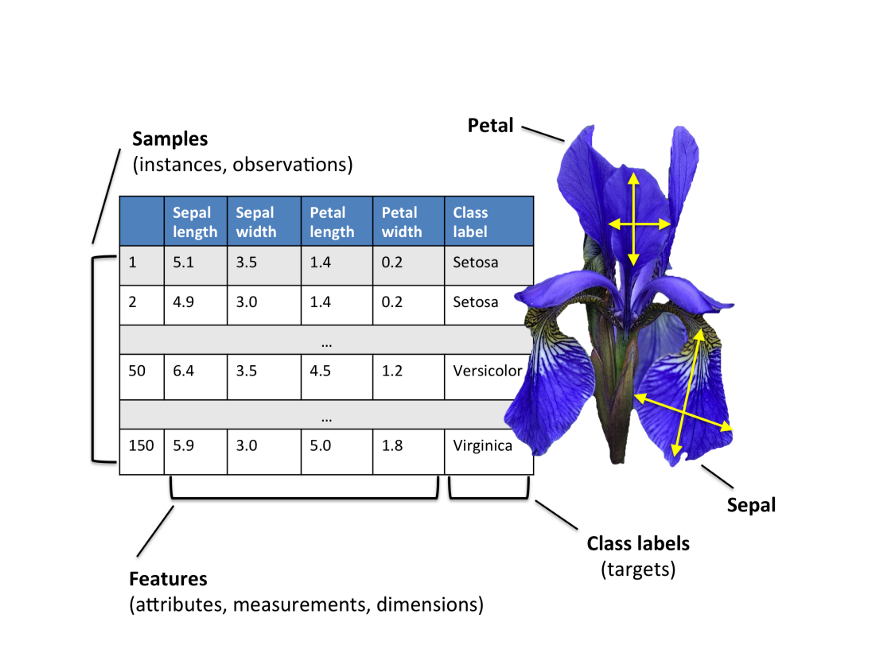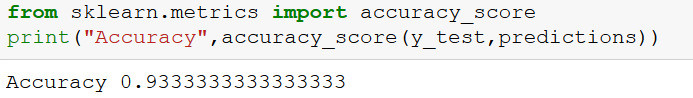Search

Updated: Feb 9

# Problem Statement

Create the model that can classify the different species of the Iris flower.

# Problem solving:

2. Build the model

3. Train the model

4. Make predictions.

# Iris Flower:

Iris is the family in the flower which contains the several species such as the iris.setosa, iris.versicolor, iris.virginica,etc.sklearn with the inbuilt datasets for the iris classification problem.

Scikit learn only works if data is stored as numeric data, irrespective of it being a regression or a classification problem. It also requires the arrays to be stored at numpy arrays for optimization. Since, this dataset is loaded from scikit learn, everything is appropriately formatted.

Let us first understand the datasets

The data set consists of:

• 150 samples

• 3 labels: species of Iris (Iris setosa, Iris virginica and Iris versicolor)

• 4 features: Sepal length, Sepal width, Petal length, Petal Width in cmpython code to load the Iris dataset.

```from sklearn import datasets

create a pandas dataframe from the iris dataset

```import pandas as pd
data=pd.DataFrame(iris['data'],columns=["Petal length","Petal Width","Sepal Length","Sepal Width"])
data["Species"] = iris["target"]```2. Analysis the iris dataset :

There are different types of plots like bar plot, box plot, scatter plot etc. Scatter plot is very useful when we are analyzing the relation ship between 2 features on x and y axis.

In seaborn library we have pairplot function which is very useful to scatter plot all the features at once instead of plotting them individually.

```import seaborn as sns
sns.pairplot(data)```We can also use histogram to analysis

```# histograms
data.hist()
plt.show()```3. Splitting the dataset

Since our process involve training and testing ,We should split our dataset. It can be executed by the following code

```x = data.drop("Species" ,axis=1)
y = data["Species"]

from sklearn.model_selection import train_test_split
x_train,x_test,y_train,y_test = train_test_split(x,y,test_size =0.3)```

x_train contains the training features

x_test contains the testing features

y_train contains the training label

y_test contains the testing labels

4. Build the Model

We can use any classification algorithm to solve the problem. but i will go with KNN

K-Nearest Neighbors (KNN)

The k-nearest neighbors (KNN) algorithm is a simple, easy-to-implement supervised machine learning algorithm that can be used to solve both classification and regression problems

```from sklearn import neighbors
classifier=neighbors.KNeighborsClassifier(n_neighbors=3)```

5. Train the Model

We can train the model with fit function.

`classifier.fit(x_train,y_train)`

Now the model is ready to make predictions

6. Make Predictions

`predictions=classifier.predict(x_test)`

Accuracy Value

predictions by our model can be matched with the expected output to measure the accuracy value.

```from sklearn.metrics import accuracy_score
print(accuracy_score(y_test,predictions))```So the Accuracy of our model is : 93.3 %

```
If you have project or assignment files, You can send at contact@codersarts.com  directly.

```

### Recent Posts

See All

#### Deploying Machine Learning Models in SageMaker - AWS Cloud.

Tel: (+91) 0120  4118730

Time :   10 : 00  AM -  08 : 00 PM IST